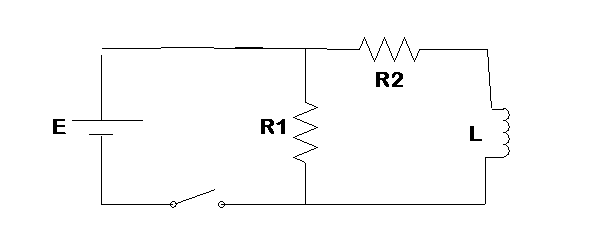# RL Circuit

Mola
I am trying to analyse an RL circuit, particulartly the dependence of current in 2 resistors with time, in an RL circuit. This is what I think but I would really love some people to tell me if I am getting it wrong or if there is anything they want to add to it.

***Just after the the switched at t = 0, the voltage in R1 is the same as the voltage in R2 because they are in parallel. Therefore the current in R1 is: I = E/R1 which is the maximum current. But the current in R2 is zero because current in the inductor is zero at t = 0.

Question:
Do I have a good reasoning for the current in the resistor R2 being zero at t = 0?

***A long time after the switched is closed: I think as time goes to infiniti, the current in R1 drops down from maximum (E/R1) to zero.
The current in R2 starts growing exponentially as time increases. And at some point the current in R2 goes steady or reach equilibrium(not growing and not decaying).

Question: Is it true that the current in R1 decays to zero as time goes to infiniti? I am not sure the current in R1 decays though because the resistor does not consume current.

I would like some help analysing this circuit. It looks pretty interesting.

Thanks, Momodou

#### Attachments

• drawing1.bmp
18 KB · Views: 465